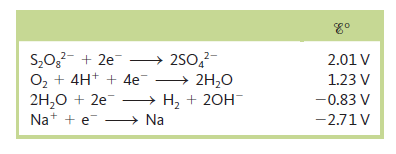# Problem: In the electrolysis of an aqueous solution of Na2SO4, what reactions occur at the anode and the cathode (assuming standard conditions)?

###### FREE Expert Solution

Na2SO4 dissociates as Naand SO42-

Na+ → gains electrons → reduction → cathode

SO42- → loses electrons → oxidation → anode

Possible cathode reactions:

2 H2O (l) + 2e- → H2 (g) + 2OH- (aq)       E = -0.83 V

Na+ (aq) + e→ Na (s)                             E = -2.71 V

100% (406 ratings)###### Problem Details

In the electrolysis of an aqueous solution of Na2SO4, what reactions occur at the anode and the cathode (assuming standard conditions)?